If Else Statement

# If Else Statement - Basics of C++ - Software Development

 Table of contentsNeed of Conditional StatementsTypes of Conditional Statements in C++if in C++Flowchart of if StatementExample of if in C++if-else in C++Flowchart of if-else StatementExample of if-elseNested if-else in C++Syntax of Nested if-elseif-else-if Ladder in C++Flowchart of if-else-if LadderSwitch Statement in C++Conditional Operator in C++Jump Statements in C++In C/C++ programming, conditional statements or decision control structures, such as if, if else, switch, etc., serve as Decision-Making Statements that enable programmers to evaluate one or more conditions and determine whether to execute a set of statements or not. These statements guide the program flow's direction by deciding which statements to execute based on the evaluated conditions.

## Need of Conditional Statements

Real-life scenarios and programming situations require decision-making. Based on the decisions made, we determine the next course of action. For instance, in C programming, we may execute a specific block of code depending on the condition. We may execute 'y' if 'x' occurs, or execute 'z' if it doesn't. Multiple conditions can also be used in programming, as in the 'else-if' statement in C. This is just one example of how to incorporate multiple conditions into programming.

## Types of Conditional Statements in C++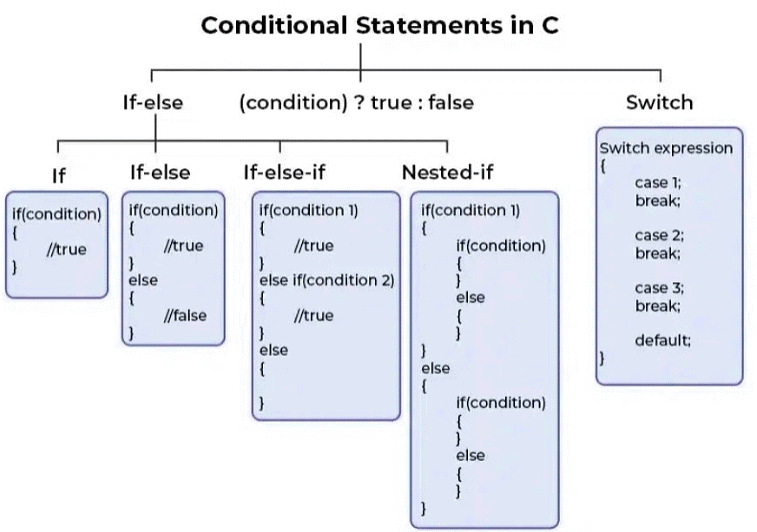Following are the decision-making statements available in C or C++:

• if Statement
• if-else Statement
• Nested if Statement
• switch Statement
• Conditional Operator
• Jump Statements:
• break
• continue
• goto
• return

## if in C++

The if statement is the fundamental decision-making statement in CC++. Its primary function is to determine whether to execute a specific statement or block of statements based on whether a particular condition is true. When a specific condition is true, a set of statements is executed; otherwise, they are not executed.
Syntax of if Statement

if(condition)

{

// Statements to execute if

// condition is true

}

Here, the condition after evaluation will be either true or false. C if statement accepts boolean values – if the value is true then it will execute the block of statements below it otherwise not. If we do not provide the curly braces ‘{‘ and ‘}’ after if(condition) then by default if statement will consider the first immediately below statement to be inside its block.

## Flowchart of if Statement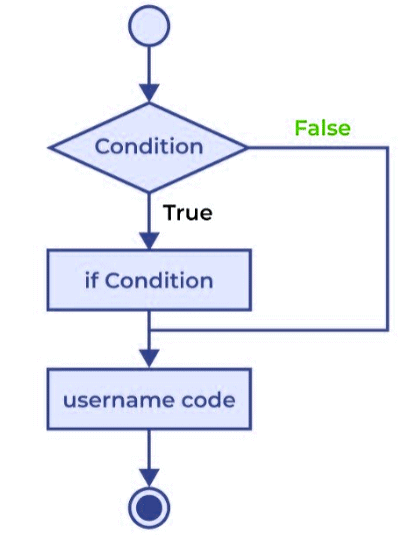## Example of if in C++

// C++ program to demonstrate if statement

#include <iostream>

using namespace std;

int main()

{

int x = 7;

if (x > 5) {

cout << "The value of x is greater than 5." << endl;

}

cout << "This line of code always executes." << endl;

return 0;

}

### Output

The value of x is greater than 5.

This line of code always executes.

• In this program, we declare an integer variable 'x' and assign it the value 7.
• We then use an if statement to check if the value of 'x' is greater than 5.
• If the condition is true, the program outputs "The value of x is greater than 5."
• Otherwise, the program skips the if block and proceeds to the next line of code.
• Finally, the program outputs "This line of code always executes." to indicate that this line of code is outside the if block and will always execute, regardless of whether the condition is true or false.

## if-else in C++

The if statement allows for the execution of a block of statements if a condition is true, but it will not execute if the condition is false. However, when there is a need to perform an action when the condition is false, the C else statement comes in handy. The else statement can be used in conjunction with the if statement to execute a block of code when the condition is false. The if-else statement is comprised of two blocks, one for the true expression and one for the false expression.

Syntax of if else in C++

if (condition)

{

// Executes this block if

// condition is true

}

else

{

// Executes this block if

// condition is false

}

## Flowchart of if-else Statement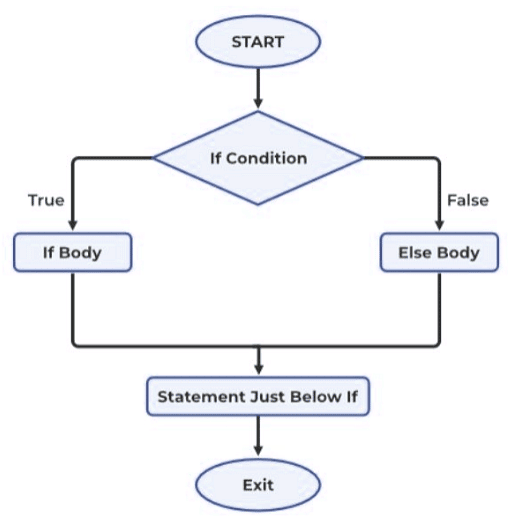## Example of if-else

#include <iostream>

using namespace std;

int main()

{

int num = 7;

if (num < 10)

cout << "The number is less than 10." << endl;

else

cout << "The number is greater than or equal to 10." << endl;

return 0;

}

### Output

The number is less than 10.

• The code declares an integer variable named "num" and assigns it a value of 7.
• The if-else statement checks whether the value of "num" is less than 10.
• If the value of "num" is less than 10, the message "The number is less than 10." is printed to the console.
• If the value of "num" is greater than or equal to 10, the message "The number is greater than or equal to 10." is printed to the console.
• Since the value of "num" is 7, which is less than 10, the output of this code is "The number is less than 10."

## Nested if-else in C++

A nested if in C is an if statement that is the target of another if statement. Nested if statements mean an if statement inside another if statement. Yes, both C and C++ allow us to nested if statements within if statements, i.e, we can place an if statement inside another if statement.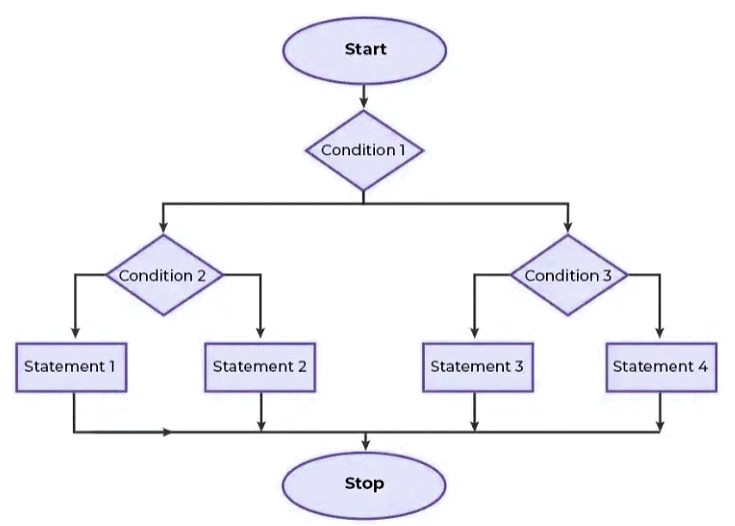## Syntax of Nested if-else

if (condition1)

{

// Executes when condition1 is true

if (condition2)

{

// Executes when condition2 is true

}

else

{

// Executes when condition2 is false

}

### Flowchart of Nested if-else

//IMAGE

Example of Nested if-else

#include <iostream>

using namespace std;

int main()

{

int age = 22;

if (age == 22) {

// First if statement

if (age < 30)

cout << "The person is younger than 30." << endl;

// Nested - if statement

// Will only be executed if

// statement above is true

if (age < 25)

cout << "The person is younger than 25 too." << endl;

else

cout << "The person is older than or equal to 25." << endl;

}

return 0;

}

### Output

The person is younger than 30.

The person is older than or equal to 25.

• The code declares an integer variable named "age" and assigns it a value of 22.
• The first if statement checks if the value of "age" is equal to 22.
• If the value of "age" is equal to 22, the nested-if statement inside the first if statement will be executed.
• The nested-if statement checks whether the value of "age" is less than 25.
• If the value of "age" is less than 25, the message "The person is younger than 25 too." is printed to the console.
• If the value of "age" is greater than or equal to 25, the message "The person is older than or equal to 25." is printed to the console.
• Since the value of "age" is 22, which is less than 30 and greater than or equal to 25, the output of this code is "The person is younger than 30. The person is older than or equal to 25."

If-else-if statements are employed when a user has to make a choice among multiple options. The execution of C if statements occurs sequentially from the top downwards. As soon as one of the conditions controlling the if is true, the associated statement is executed, and the rest of the else-if ladder is bypassed. If none of the conditions is true, then the final else statement will be executed. The if-else-if ladder shares similarities with the switch statement.

if (condition)

statement;

else if (condition)

statement;

.

.

else

statement;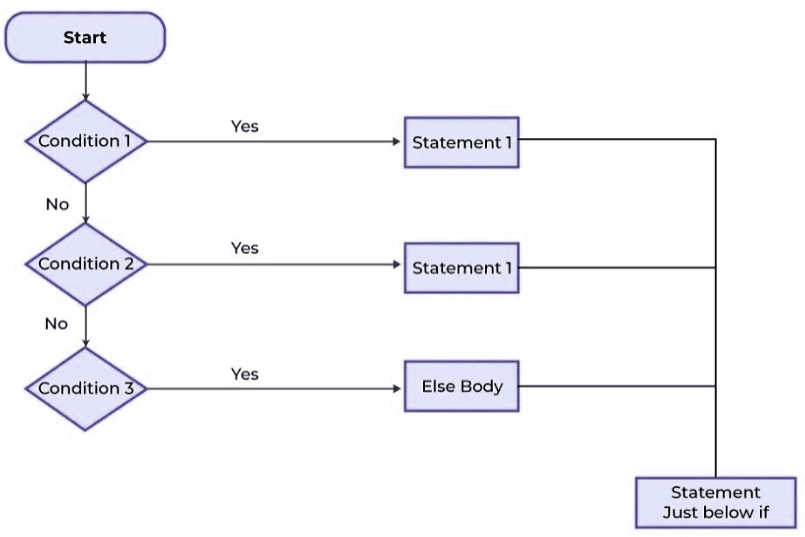#include <iostream>

using namespace std;

int main()

{

int num = 35;

if (num < 20)

cout << "The number is less than 20." << endl;

else if (num < 30)

cout << "The number is between 20 and 29." << endl;

else if (num < 40)

cout << "The number is between 30 and 39." << endl;

else

cout << "The number is greater than or equal to 40." << endl;

return 0;

}

### Output

The number is between 30 and 39.

• The code declares an integer variable named "num" and assigns it a value of 35.
• The if-else-if ladder checks whether the value of "num" satisfies any of the conditions specified.
• The first if statement checks if the value of "num" is less than 20. If true, the message "The number is less than 20." is printed to the console.
• The else-if statement checks if the value of "num" is between 20 and 29 (i.e., less than 30). If true, the message "The number is between 20 and 29." is printed to the console.
• The second else-if statement checks if the value of "num" is between 30 and 39. If true, the message "The number is between 30 and 39." is printed to the console.
• If none of the conditions are true, the final else statement is executed and the message "The number is greater than or equal to 40." is printed to the console.
• Since the value of "num" is 35, which is between 30 and 39, the output of this code is "The number is between 30 and 39."

## Switch Statement in C++

The switch case statement provides an alternative to the if-else-if ladder and is used to execute conditional code based on the value of the variable specified in the switch statement. The switch block consists of cases that are executed based on the value of the switch variable.

Syntax of switch

switch (expression) {

case value1:

statements;

case value2:

statements;

....

....

....

default:

statements;

}

### Flowchart of switch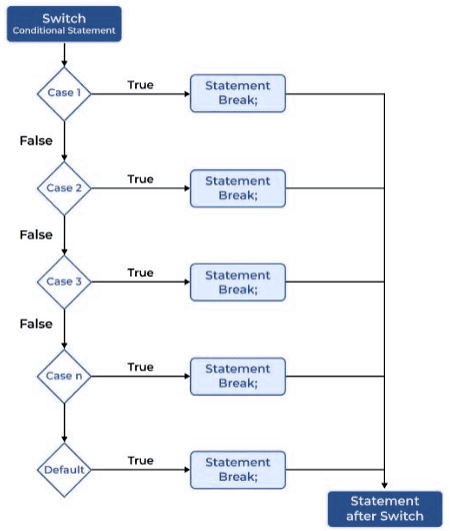### Example of switch Statement

// C++ program to illustrate the use of switch statement

#include <iostream>

using namespace std;

int main()

{

int num = 3;

switch(num) {

case 1:

cout << "The number is 1";

break;

case 2:

cout << "The number is 2";

break;

case 3:

cout << "The number is 3";

break;

default:

cout << "The number is not in the switch cases";

break;

}

return 0;

}

The number is 3

## Conditional Operator in C++

The conditional operator is a programming tool used to add conditional code to our programs. It functions similarly to the if-else statement, and is sometimes called the ternary operator because it operates on three operands.

Syntax of Conditional Operator

(condition) ? [true_statements] : [flase_statements];

### Flowchart of Conditional Operator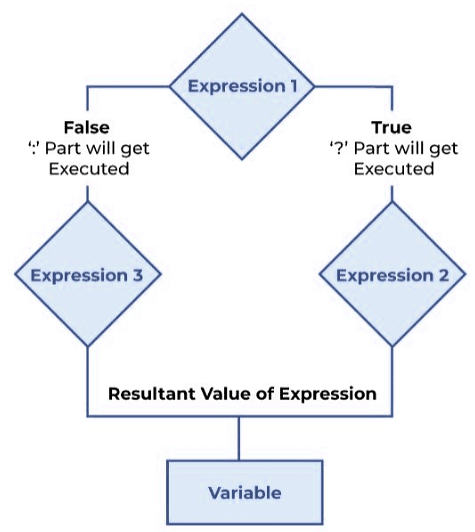### Example of Conditional Operator

// C++ Program to illustrate the use of conditional operator

#include <iostream>

using namespace std;

// driver code

int main()

int var;

int flag = 0;

// using conditional operator to assign the value to var

// according to the value of flag

var = flag == 0 ? 25 : -25;

cout << "Value of var when flag is 0: " << var << endl;

// changing the value of flag

flag = 1;

// again assigning the value to var using same statement

var = flag == 0 ? 25 : -25;

cout << "Value of var when flag is NOT 0: " << var;

return 0;

}

### Output

Value of var when flag is 0: 25

Value of var when flag is NOT 0: -25

## Jump Statements in C++

These statements are used in C or C++ for the unconditional flow of control throughout the functions in a program. They support four types of jump statements:

### break

This loop control statement is used to terminate the loop. As soon as the break statement is encountered from within a loop, the loop iterations stop there, and control returns from the loop immediately to the first statement after the loop.
Syntax of break

break;

Basically, break statements are used in situations when we are not sure about the actual number of iterations for the loop or we want to terminate the loop based on some condition.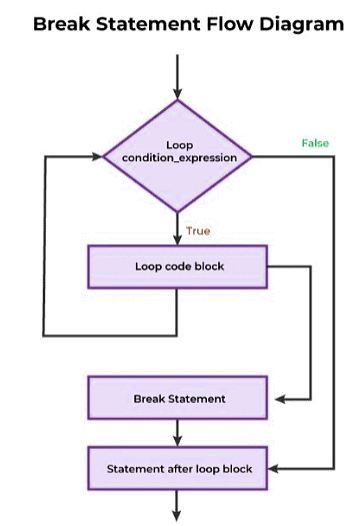### Example of break

// C++ program to demonstrate the usage of the break statement

#include <iostream>

using namespace std;

void searchElement(int arr[], int size, int target)

{

// Loop to traverse the array and search for the target element

for (int i = 0; i < size; i++) {

if (arr[i] == target) {

cout << "Element found at position: " << (i + 1);

break;

}

}

}

// Driver program to test the above function

int main()

{

int array[] = { 10, 20, 30, 40, 50 };

int length = 5; // number of elements in the array

int target = 30; // target element to be searched

// Calling the function to find the target element

searchElement(array, length, target);

return 0;

}

• This C++ program demonstrates the usage of the break statement. The program defines a function called 'searchElement' that takes an array, its size, and a target element as arguments.
• Within the function, there is a loop that traverses the array and checks if each element is equal to the target element. If a match is found, it prints the position of the element and breaks out of the loop using the break statement.
• In the main function, an array named 'array' is defined with some elements. The length of the array is set to 5, and the target element is set to 30.
• The 'searchElement' function is then called with the array, its length, and the target element as arguments.
• If the target element is found in the array, the program prints the position of the element. If the target element is not present, nothing is printed.
• Finally, the program terminates and returns 0.

### Continue

The continue statement in C++ is similar to the break statement, but has an opposite effect. While the break statement terminates the loop, the continue statement forces the next iteration of the loop to execute. When the continue statement is encountered within a loop, it causes the code following the continue statement to be skipped, and the next iteration of the loop to begin. This means that any statements following the continue statement within the current iteration will not be executed, but the loop will continue to execute.

Syntax of continue

continue;

Flowchart of Continue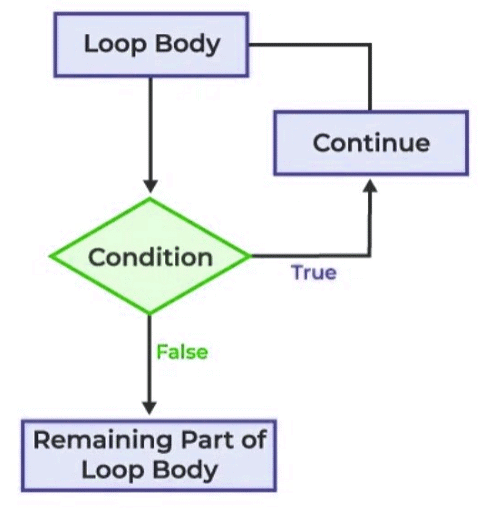Example of continue

// C++ program to explain the use

// of continue statement

#include <iostream>

using namespace std;

int main()

{

// loop from 1 to 10

for (int i = 1; i <= 10; i++) {

// If i is equals to 6,

// continue to next iteration

// without printing

if (i == 6)

continue;

else

// otherwise print the value of i

cout << i << " ";

}

return 0;

}

### Output

1 2 3 4 5 7 8 9 10

The document If Else Statement | Basics of C++ - Software Development is a part of the Software Development Course Basics of C++.
All you need of Software Development at this link: Software Development

## Basics of C++

70 videos|45 docs|15 tests

## Basics of C++

70 videos|45 docs|15 testsExplore Courses for Software Development examSignup to see your scores go up within 7 days! Learn & Practice with 1000+ FREE Notes, Videos & Tests.
10M+ students study on EduRev
Track your progress, build streaks, highlight & save important lessons and more!
Related Searches

,

,

,

,

,

,

,

,

,

,

,

,

,

,

,

,

,

,

,

,

,

;